2020/02/24 22:07

#下载安装命令

## CPU版本安装命令
pip install -f https://paddlepaddle.org.cn/pip/oschina/cpu paddlepaddle

## GPU版本安装命令
pip install -f https://paddlepaddle.org.cn/pip/oschina/gpu paddlepaddle-gpu

# 01 导读

1. 深度学习基础知识

2. numpy实现神经网络构建和梯度下降算法

3. 计算机视觉领域主要方向的原理、实践

4. 自然语言处理领域主要方向的原理、实践

5. 个性化推荐算法的原理、实践

## 02 目标检测概述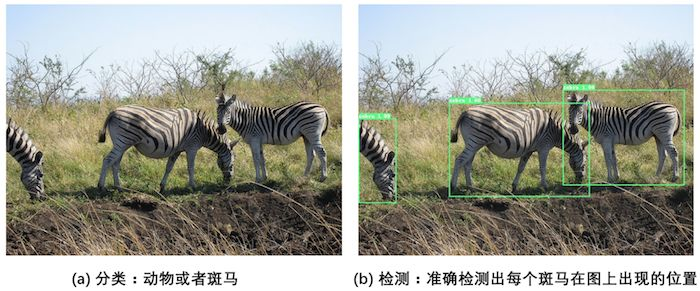• 图1（a）是图像分类任务，只需识别出这是一张斑马的图片。

• 图1（b）是目标检测任务，不仅要识别出这是一张斑马的图片，还要标出图中斑马的位置。

## 03 目标检测发展历程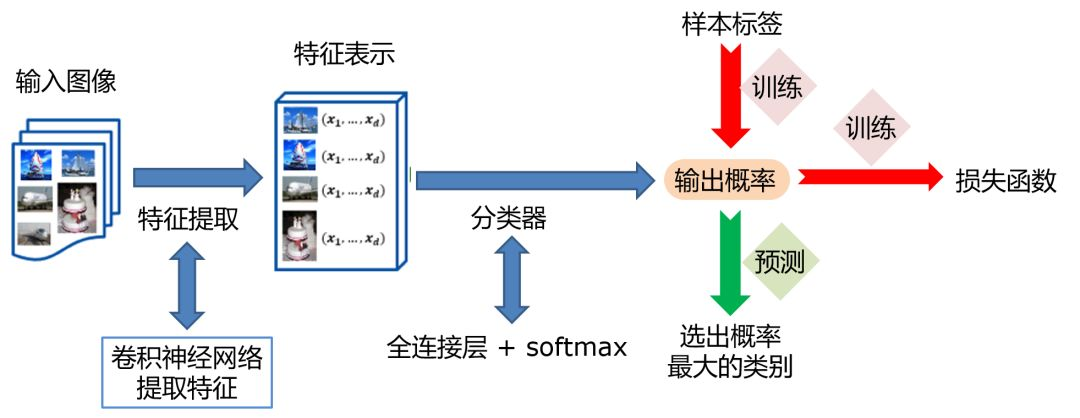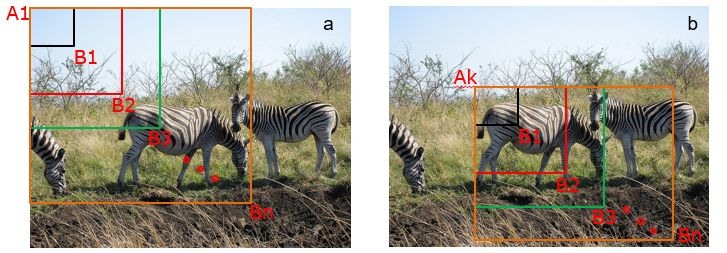A为图像上的某个像素点，B为A右下方另外一个像素点，A、B两点可以确定一个矩形框，记作AB。

• 如图3（a）所示：A在图片左上角位置，B遍历除A之外的所有位置，生成矩形框A1B1, …, A1Bn, …

• 如图3（b）所示：A在图片中间某个位置，B遍历A右下方所有位置，生成矩形框AkB1, …, AkBn, …

• 2013年，Ross Girshick 等人于首次将CNN的方法应用在目标检测任务上，他们使用传统图像算法selective search产生候选区域，取得了极大的成功，这就是对目标检测领域影响深远的区域卷积神经网络(R-CNN)模型。

• 2015年，Ross Girshick 对此方法进行了改进，提出了Fast RCNN模型。通过将不同区域的物体共用卷积层的计算，大大缩减了计算量，提高了处理速度，而且还引入了调整目标物体位置的回归方法，进一步提高了位置预测的准确性。

• 2015年，Shaoqing Ren 等人提出了Faster RCNN模型，提出了RPN的方法来产生物体的候选区域，这一方法里面不再需要使用传统的图像处理算法来产生候选区域，进一步提升了处理速度。

• 2017年，Kaiming He 等人于提出了Mask RCNN模型，只需要在Faster RCNN模型上添加比较少的计算量，就可以同时实现目标检测和物体实例分割两个任务。

R-CNN的系列算法分成两个阶段，先在图像上产生候选区域，再对候选区域进行分类并预测目标物体位置，它们通常被叫做两阶段检测算法。SSD和YOLO算法则只使用一个网络同时产生候选区域并预测出物体的类别和位置，所以它们通常被叫做单阶段检测算法。由于篇幅所限，本章将重点介绍YOLO-V3算法，并用其完成林业病虫害数据集中的昆虫检测任务，主要涵盖如下内容：

• 图像检测基础概念：介绍与目标检测任相关的基本概念，包括边界框、锚框和交并比等。

• 林业病虫害数据集：介绍数据集结构及数据预处理方法。

• YOLO-V3目标检测模型：介绍算法原理，及如何应用林业病虫害数据集进行模型训练和测试。

## 04 目标检测基础概念1. xyxy，即，其中是矩形框左上角的坐标，是矩形框右下角的坐标。图4中3个红色矩形框用xyxy格式表示如下：
• 左：。

• 中：。

• 右：。

1. xywh，即，其中是矩形框中心点的坐标，w是矩形框的宽度，h是矩形框的高度。

1. 在阅读代码时，请注意使用的是哪一种格式的表示方式。

2. 图片坐标的原点在左上角，x轴向右为正方向，y轴向下为正方向。

### 锚框（Anchor）

# 画图展示如何绘制边界框和锚框
import numpy as np
import matplotlib.pyplot as plt
import matplotlib.patches as patches
from matplotlib.image import imread
import math

# 定义画矩形框的程序
def draw_rectangle(currentAxis, bbox, edgecolor = 'k', facecolor = 'y', fill=False, linestyle='-'):
# currentAxis，坐标轴，通过plt.gca()获取
# bbox，边界框，包含四个数值的list， [x1, y1, x2, y2]
# edgecolor，边框线条颜色
# facecolor，填充颜色
# fill, 是否填充
# linestype，边框线型
# patches.Rectangle需要传入左上角坐标、矩形区域的宽度、高度等参数
rect=patches.Rectangle((bbox, bbox), bbox-bbox+1, bbox-bbox+1, linewidth=1,
edgecolor=edgecolor,facecolor=facecolor,fill=fill, linestyle=linestyle)
currentAxis.add_patch(rect)

plt.figure(figsize=(10, 10))

filename = '/home/aistudio/work/images/section3/000000086956.jpg'
im = imread(filename)
plt.imshow(im)

# 使用xyxy格式表示物体真实框
bbox1 = [214.29, 325.03, 399.82, 631.37]
bbox2 = [40.93, 141.1, 226.99, 515.73]
bbox3 = [247.2, 131.62, 480.0, 639.32]

currentAxis=plt.gca()

draw_rectangle(currentAxis, bbox1, edgecolor='r')
draw_rectangle(currentAxis, bbox2, edgecolor='r')
draw_rectangle(currentAxis, bbox3,edgecolor='r')

# 绘制锚框
def draw_anchor_box(center, length, scales, ratios, img_height, img_width):
"""
以center为中心，产生一系列锚框
其中length指定了一个基准的长度
scales是包含多种尺寸比例的list
ratios是包含多种长宽比的list
img_height和img_width是图片的尺寸，生成的锚框范围不能超出图片尺寸之外
"""
bboxes = []
for scale in scales:
for ratio in ratios:
h = length*scale*math.sqrt(ratio)
w = length*scale/math.sqrt(ratio)
x1 = max(center - w/2., 0.)
y1 = max(center - h/2., 0.)
x2 = min(center + w/2. - 1.0, img_width - 1.0)
y2 = min(center + h/2. - 1.0, img_height - 1.0)
print(center, center, w, h)
bboxes.append([x1, y1, x2, y2])

for bbox in bboxes:
draw_rectangle(currentAxis, bbox, edgecolor = 'b')

img_height = im.shape
img_width = im.shape
draw_anchor_box([300., 500.], 100., [2.0], [0.5, 1.0, 2.0], img_height, img_width)

################# 以下为添加文字说明和箭头###############################

plt.text(285, 285, 'G1', color='red', fontsize=20)
plt.arrow(300, 288, 30, 40, color='red', width=0.001, length_includes_head=True, \
head_width=5, head_length=10, shape='full')

plt.text(190, 320, 'A1', color='blue', fontsize=20)
plt.arrow(200, 320, 30, 40, color='blue', width=0.001, length_includes_head=True, \
head_width=5, head_length=10, shape='full')

plt.text(160, 370, 'A2', color='blue', fontsize=20)
plt.arrow(170, 370, 30, 40, color='blue', width=0.001, length_includes_head=True, \
head_width=5, head_length=10, shape='full')

plt.text(115, 420, 'A3', color='blue', fontsize=20)
plt.arrow(127, 420, 30, 40, color='blue', width=0.001, length_includes_head=True, \
head_width=5, head_length=10, shape='full')

#draw_anchor_box([200., 200.], 100., [2.0], [0.5, 1.0, 2.0])
plt.show()


(300.0, 500.0, 282.84271247461896, 141.4213562373095)
(300.0, 500.0, 200.0, 200.0)
(300.0, 500.0, 141.42135623730948, 282.842712474619)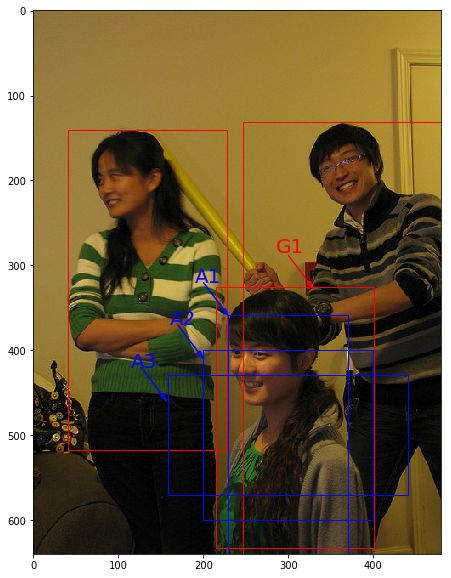### 交并比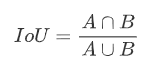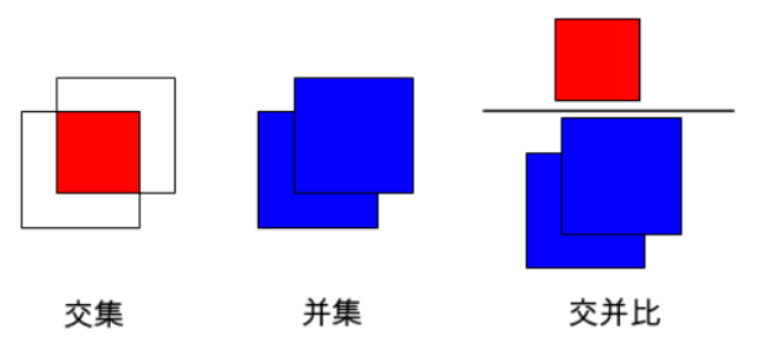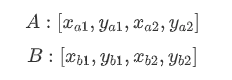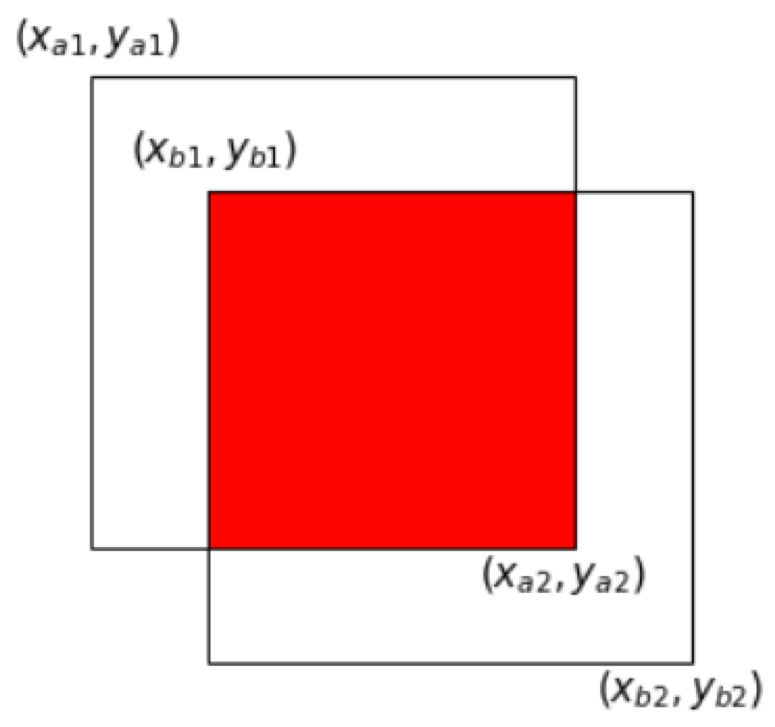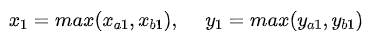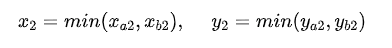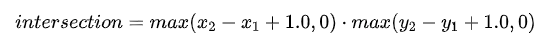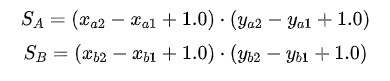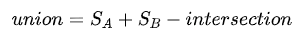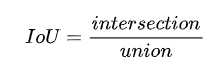# 计算IoU，矩形框的坐标形式为xyxy，这个函数会被保存在box_utils.py文件中
def box_iou_xyxy(box1, box2):
# 获取box1左上角和右下角的坐标
x1min, y1min, x1max, y1max = box1, box1, box1, box1
# 计算box1的面积
s1 = (y1max - y1min + 1.) * (x1max - x1min + 1.)
# 获取box2左上角和右下角的坐标
x2min, y2min, x2max, y2max = box2, box2, box2, box2
# 计算box2的面积
s2 = (y2max - y2min + 1.) * (x2max - x2min + 1.)

# 计算相交矩形框的坐标
xmin = np.maximum(x1min, x2min)
ymin = np.maximum(y1min, y2min)
xmax = np.minimum(x1max, x2max)
ymax = np.minimum(y1max, y2max)
# 计算相交矩形行的高度、宽度、面积
inter_h = np.maximum(ymax - ymin + 1., 0.)
inter_w = np.maximum(xmax - xmin + 1., 0.)
intersection = inter_h * inter_w
# 计算相并面积
union = s1 + s2 - intersection
# 计算交并比
iou = intersection / union
return iou

bbox1 = [100., 100., 200., 200.]
bbox2 = [120., 120., 220., 220.]
iou = box_iou_xyxy(bbox1, bbox2)
print('IoU is {}'.format(iou))


IoU is 0.474026443176



# 计算IoU，矩形框的坐标形式为xywh
def box_iou_xywh(box1, box2):
x1min, y1min = box1 - box1/2.0, box1 - box1/2.0
x1max, y1max = box1 + box1/2.0, box1 + box1/2.0
s1 = box1 * box1

x2min, y2min = box2 - box2/2.0, box2 - box2/2.0
x2max, y2max = box2 + box2/2.0, box2 + box2/2.0
s2 = box2 * box2

xmin = np.maximum(x1min, x2min)
ymin = np.maximum(y1min, y2min)
xmax = np.minimum(x1max, x2max)
ymax = np.minimum(y1max, y2max)
inter_h = np.maximum(ymax - ymin, 0.)
inter_w = np.maximum(xmax - xmin, 0.)
intersection = inter_h * inter_w

union = s1 + s2 - intersection
iou = intersection / union
return iou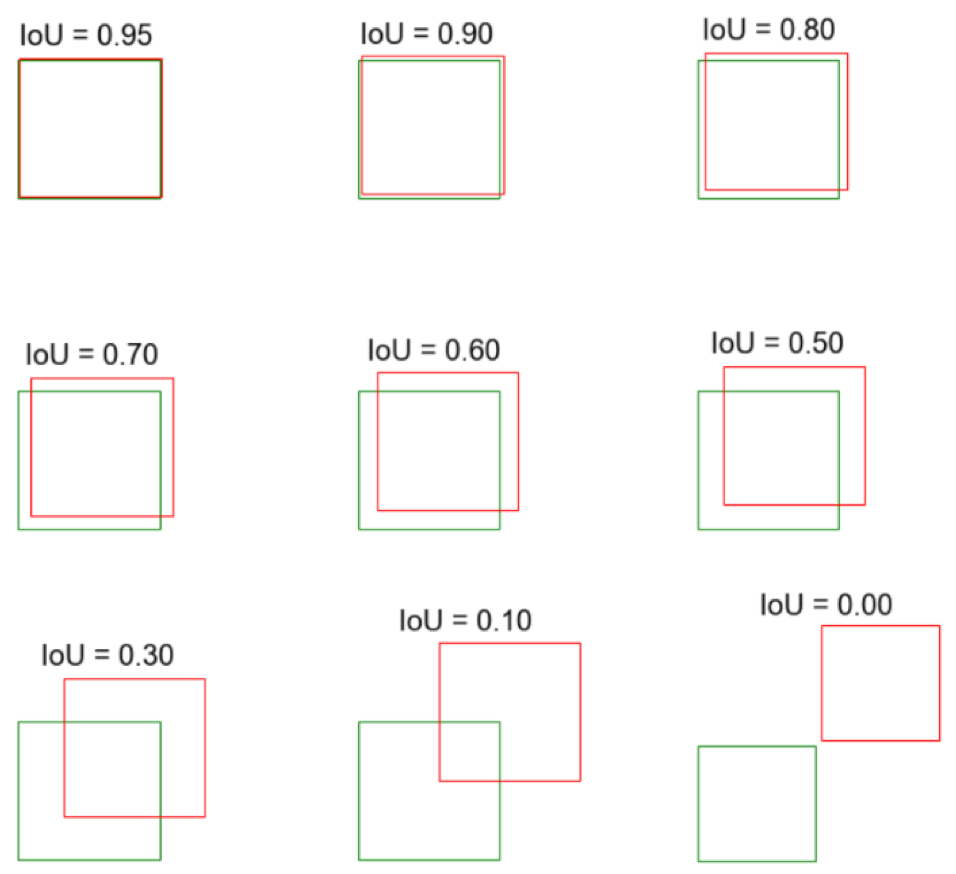05 总结

【如何学习】

1 如何观看配套视频？如何代码实践？

https://aistudio.baidu.com/aistudio/course/introduce/888

2 学习过程中，有疑问怎么办？

3 如何学习更多内容？

下载安装命令

## CPU版本安装命令
pip install -f https://paddlepaddle.org.cn/pip/oschina/cpu paddlepaddle

## GPU版本安装命令
pip install -f https://paddlepaddle.org.cn/pip/oschina/gpu paddlepaddle-gpu>> 访问 PaddlePaddle 官网，了解更多相关内容

0
0 收藏

### 作者的其它热门文章0 评论
0 收藏
0